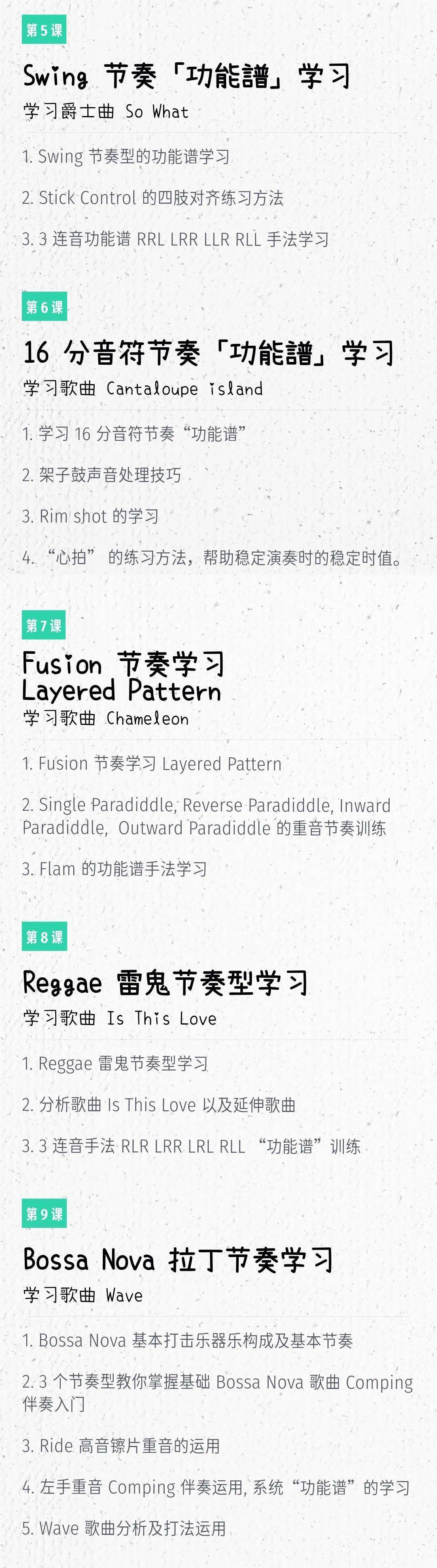# 【爵士鼓】学会这个节奏，马上开始演奏爵士乐！

Swing摇摆分家练习

Ride的重音转换

So What

/ Swing摇摆分家练习 /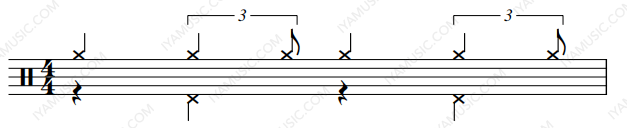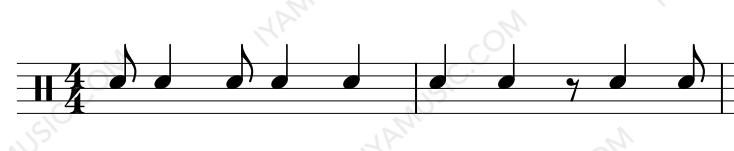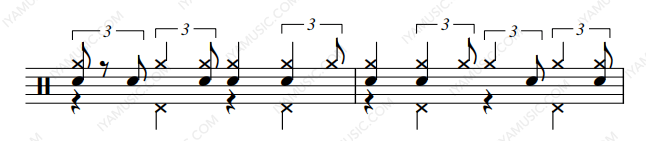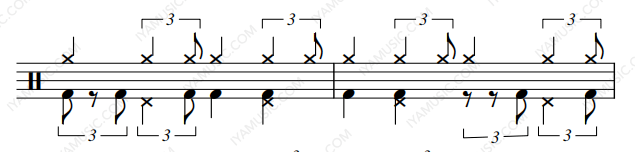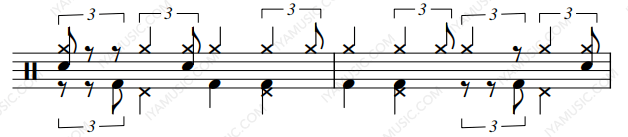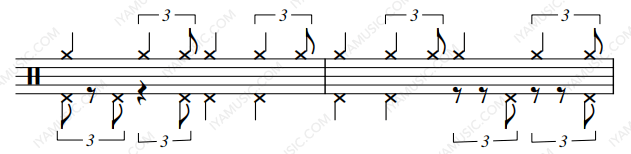1、R L R L

2、L R L R

L=军鼓 R=底鼓

R L R L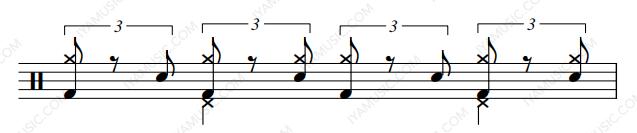L R L R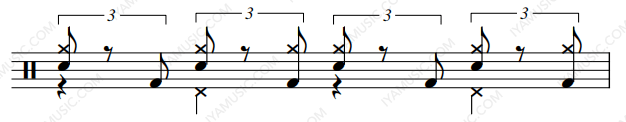L=踩镲 R=军鼓

R L R L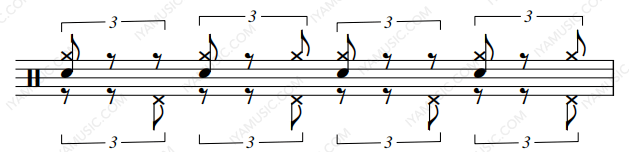L R L R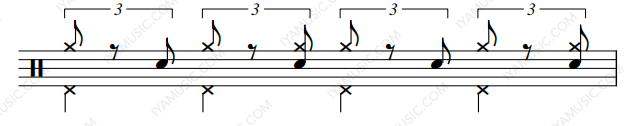L=踩镲 R=底鼓

R L R L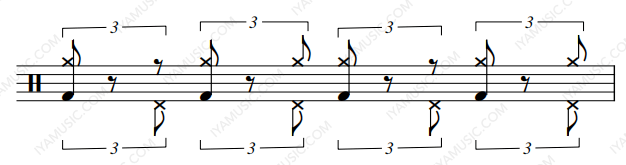L R L R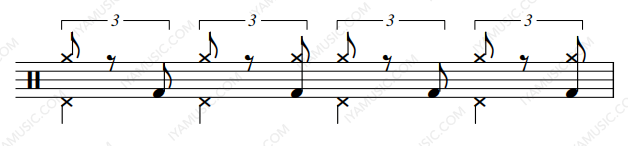/ Ride的重音转换 /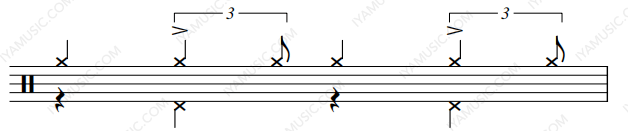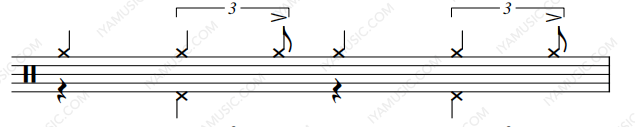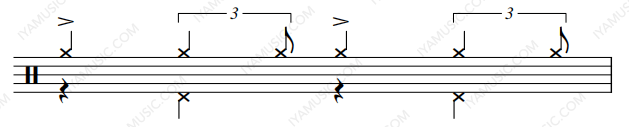/ So What /